# Introduction to the mathematical principle of convolutional neural networks

Share
/ 2019-04-22 / News

[Introduction] Convolutional neural networks have become an important part of the development of computer neuro-visual technology. This paper introduces the mathematical principles of convolutional neural networks. . The article includes four main contents: convolution, convolutional layer, pooling layer and the principle of back propagation in convolutional neural networks. In the introduction to the convolution section, the author introduces the definition of convolution, efficient convolution and the same convolution, stride convolution, and 3D convolution. In the convolutional layer section, the authors describe the role of connection cutting and parameter sharing in reducing the amount of network parameter learning. In the pooling layer, the author introduces the meaning of pooling and the use of masks.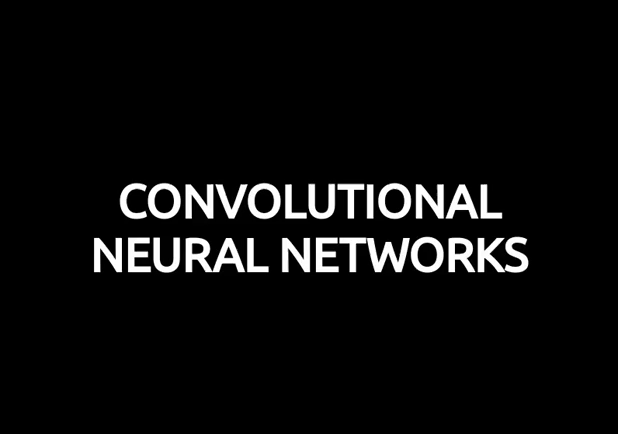Automated driving, smart medical care, smart retailing, things that were once thought impossible,With the help of computer vision technology, it has finally become a reality. Today, the dream of autonomous driving and automated grocery stores is no longer as far away as it used to be. In fact, every day we use computer vision technology to help us unlock your phone with a human face, or to automatically retouch photos that are about to be posted on social media. Behind the great success of computer vision technology applications, convolutional neural networks (CNN) are probably the most important component. In this article, we will gradually understand how neural networks work with CNN-specific ideas. This article contains quite complex mathematical equations, but don't be discouraged if you are unfamiliar with linear algebra and calculus. My goal is not to let you remember those formulas, but to let you intuitively understand the hidden meaning behind these formulas.

Foreword

In the previous series, we learned about densely connected neural networks ( Densely connected neural networks). The neurons of these networks are divided into groups.A continuous layer is formed, and neurons between adjacent two layers are connected to each other. The figure below shows an example of a densely connected neural network.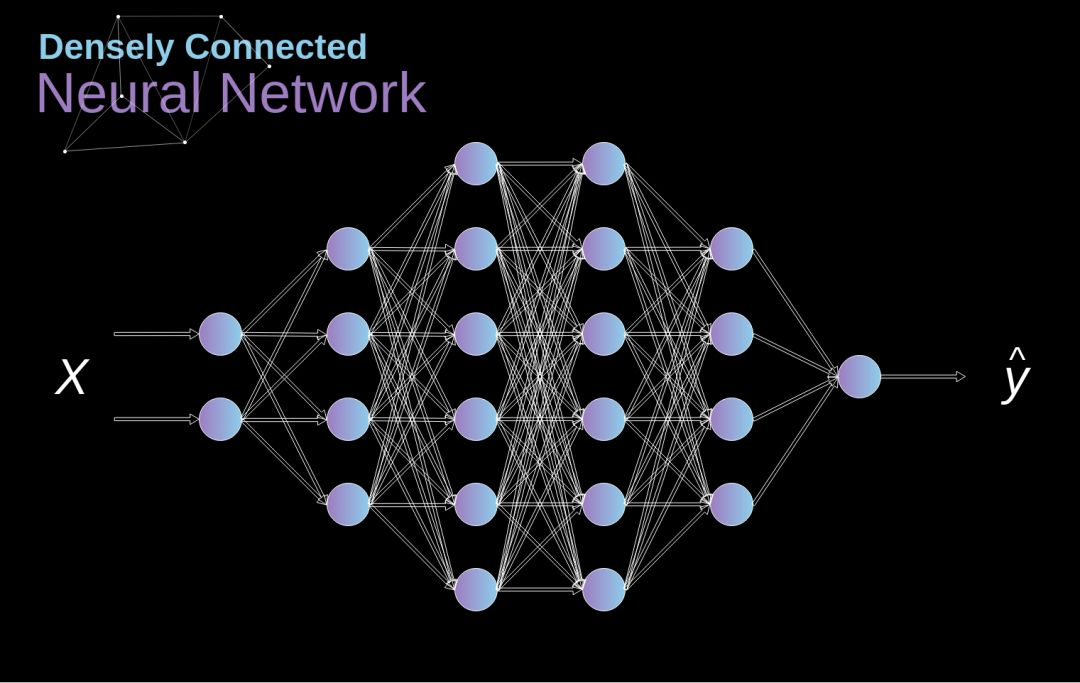Figure 1. Densely connected neural network architecture

When we solve the classification problem, if our feature is a limited set And there are well-defined features, this method is very effective &mdash;&mdash; for example, based on the statistics recorded by football players during the game, predict the position of the athlete. However, when using photos to make predictions, the situation becomes more complicated.We can of course treat the brightness of each pixel as a separate feature and pass it as input to our dense network. Unfortunately, in order for a neural network to handle typical smartphone photos, the network must contain tens of millions or even hundreds of millions of neurons. We can also process phone photos by reducing the size of the photo, but doing so will cause us to lose a lot of valuable information. It can be found that the performance of this traditional strategy is very poor, so we need a new, smarter way to use the data as much as possible while reducing the number of calculations and parameters necessary. It’s time for CNN to debut.

Data Structure of Digital Image

First take a moment to explain the storage of digital images the way. Digital images are actually huge digital matrices. Each number in the matrix corresponds to the brightness of its pixels. In the RGB model, the color image consists of three matrices, corresponding to three color channels &mdash; &mdash; red, green,blue. In black and white images, we only need one matrix. Each number in the matrix has a value range of 0 to 255. This range is a compromise between the efficiency of storing image information (256 values just fit 1 byte) and the sensitivity of the human eye (we distinguish the number of gray levels of the same color).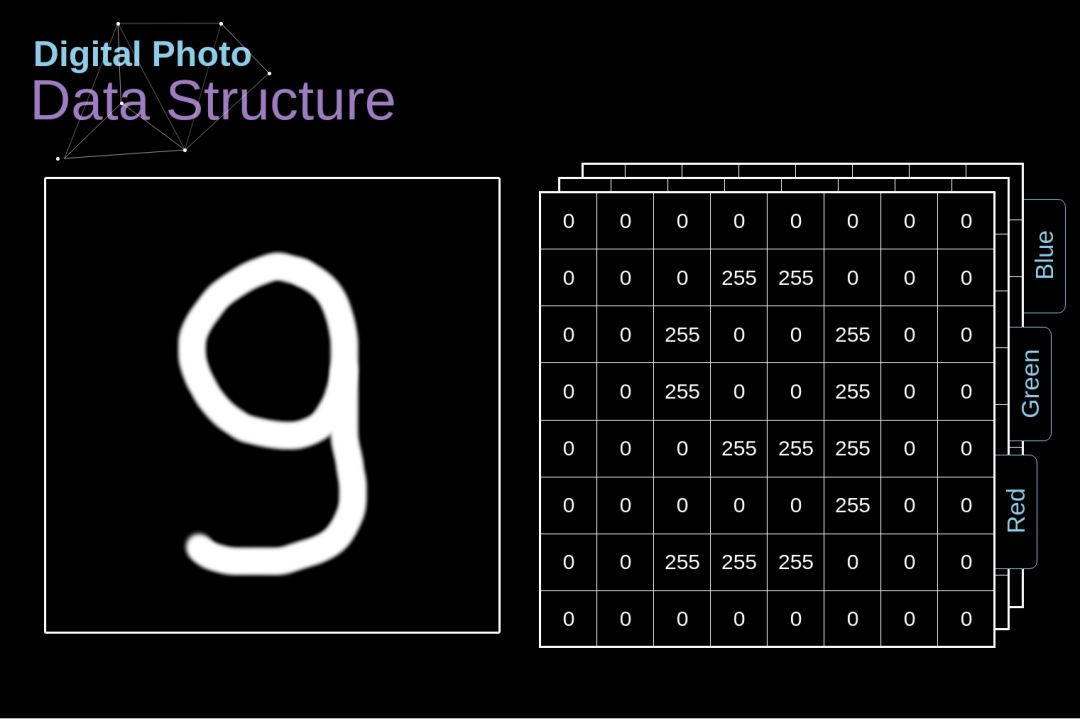Figure 2. Data structure behind digital images

convolution

kernel convolution is not just for CNN,It is also a key element of many other computer vision algorithms. Nuclear convolution is the process of sliding a small digital matrix (filter, also known as kernel or filter) onto an image and transforming the value of the image matrix based on the value of the kernel. The output obtained after the image has been subjected to a convolution operation is called a feature map. The value of the feature map is calculated as follows, where f represents the input image and h represents the filter. The number of rows and columns of the result matrix are represented by m and n, respectively.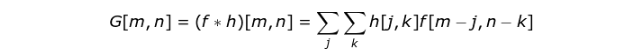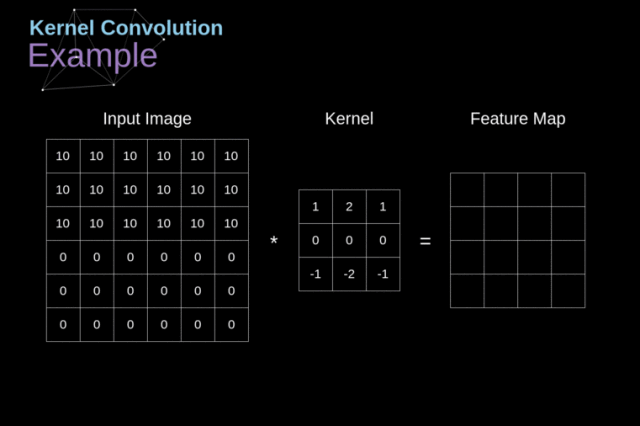Figure 3. Kernel convolution example

After placing the kernel on the selected pixel, we take each value from the kernel in turn and multiply them by the corresponding values in the image Finally, we add the result elements of each kernel operation and place the summation result in the correct position in the output feature map. The above figure shows the process of this operation in detail from the microscopic point, but in the complete The result of implementing this operation on the image may be more interesting. Figure 4 shows the convolution results using several different kernels.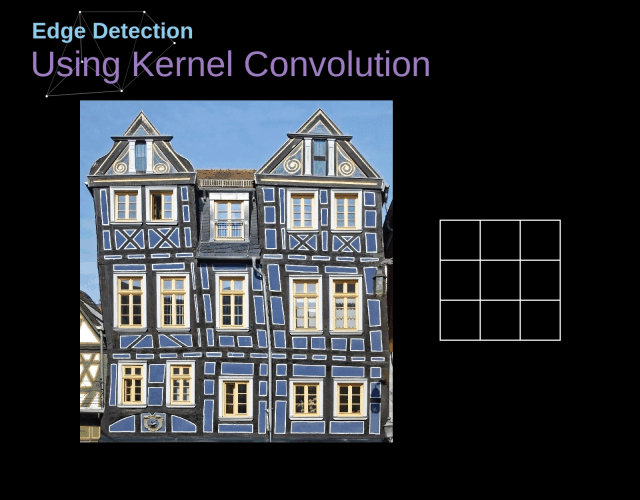Figure 4. Finding Edges with kernel convolution

Valid and Same Convolution

As we saw in Figure 3, when we use a 3x3 kernel to perform convolution on a 6x6 image, we get a 4x4 feature map. This is because in this image, only 16 positions can complete kerenl Place it in this image.Since our image shrinks each time we perform a convolution, we can only make a limited number of convolutions before our image completely disappears. In addition, if we observe the process of moving the kernel in the image, we will find that the influence of the pixels on the periphery of the image is much smaller than the influence of the pixels in the center of the image. This will cause us to lose some of the information contained in the image. The figure below shows the effect of changes in pixel position on the feature map.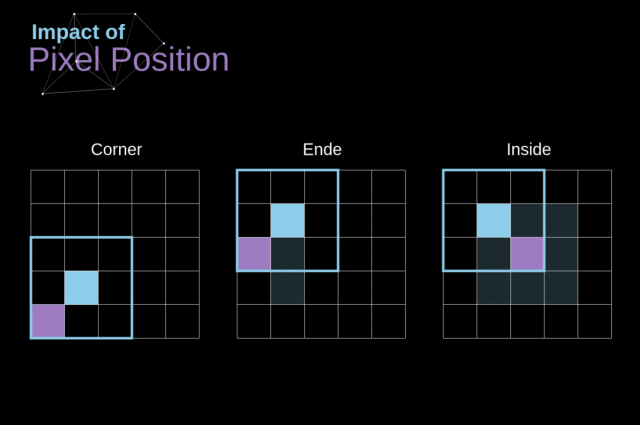Figure 5. Impact of pixel position

In order to solve these two problems,We can use an extra border to fill the image (padding). For example, if you use 1 pixel for padding, we increase the size of the image to 8x8, so with a convolution of the 3x3 kernel, the output size will be 6x6. In practice, we usually fill in extra boundaries with zero values. Depending on whether or not to use padding, we will handle two types of convolution &mdash;&mdash;Valid and Same. Valid&mdash;&mdash;Use the original image, Same&mdash;&mdash;Use the original image and use the border around it to make the input and output images the same size. In the second case, the fill width should satisfy the following equation, where p is the fill size and f is the kernel size (usually an odd number).

Stranded Convolution )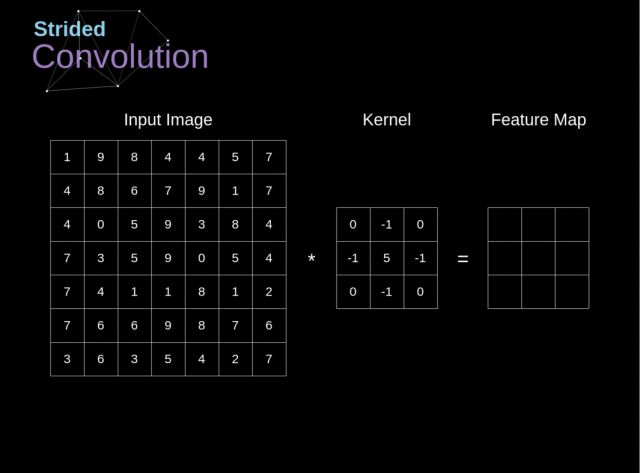Figure 6. Example of strided convolution

In the previous example, We always move the kernel one pixel at a time, with a step size of 1.The step size can also be considered as one of the hyperparameters of the convolutional layer. Figure 6 shows the convolution operation when using a larger step size. When designing the CNN architecture, we can increase the step size if we want less perceptual domain overlap or if we want the spatial dimension of the feature map to be smaller. The size of the output matrix (when considering fill and step size) can be calculated using the following formula.Transition to the third dimension

Convolution over volume is a very important concept that not only allows us to process color images,And more importantly, we can use multiple kernels in a single-layer network. The first rule is that the kernel and the image must have the same number of channels. In general, the processing of the image is very similar to the example of Figure 3, but this time we are multiplying the pairs of values in the three-dimensional space. If you want to use multiple kernels on the same image, first we need to perform a convolution on each kernel separately, then stack the results down from the top level, and finally combine them into a whole. The size of the output tensor (which can be called a 3D matrix) satisfies the following equation, where: n - image size, f - filter size, nc - number of channels in the image, p - fill size, s - stride size, nf - The number of kernels.

Convolutional layer

Next we will use the knowledge we have learned to build a layer of CNN. The method we will use is almost as strong as constructing the nerve. The same is true when using the network. The only difference is that we no longer use simple matrix multiplication, but instead use convolution. Forward propagation consists of two steps. The first step is to calculate the intermediate value Z: first the previous one The input data of the layer is convolved with the tensor W (including the filter), and then the result of the operation is added with the deviation b. The second step is to input the intermediate value Z into the nonlinear activation function (using g to represent the activation function) The mathematical formula in matrix form is shown below. If you are not clear about any part of the formula,I highly recommend reading the previous article, which details the details of the densely connected neural network. The illustration below shows the dimensions of the tensors in the formula to aid understanding.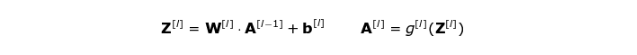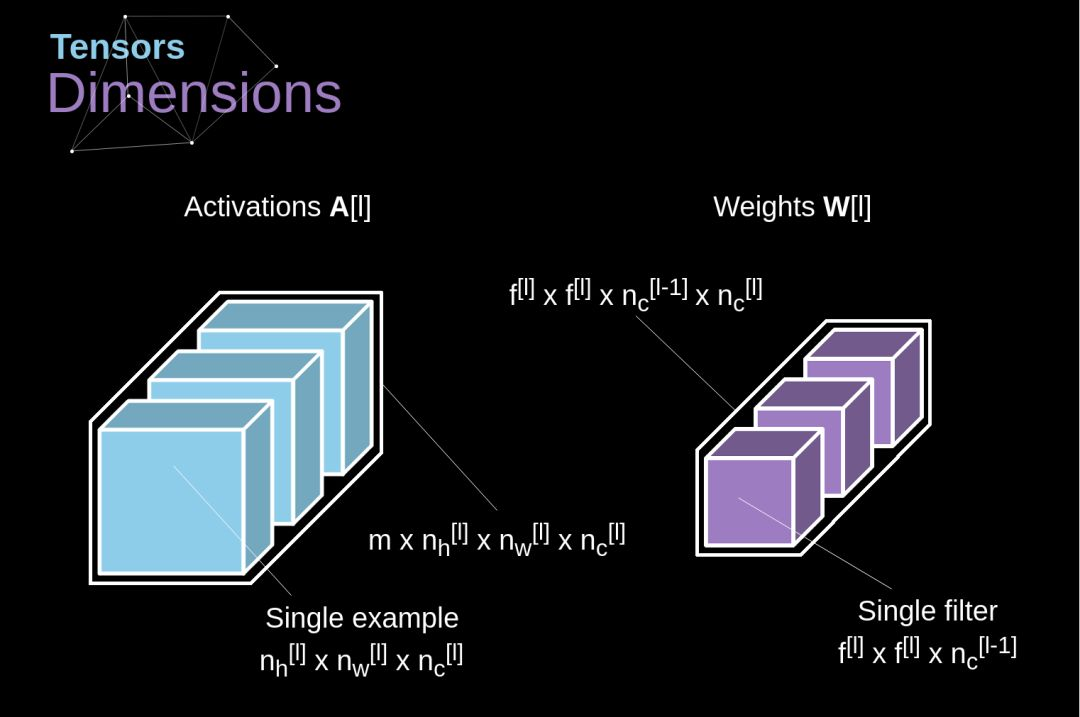Figure 8. Tensors dimensions

Connections Cutting and Parameters Sharing

In this article As mentioned at the beginning, due to the need to learn a large number of parameters, the densely connected neural network has a poor ability to process images, and convolution provides a solution to this problem. Let's take a look at the convolution. How to optimize the calculation of image processing. In the following figure, we visualize 2D convolution in a slightly different way &mdash; neurons labeled with numbers 1-9 form the input layer for receiving pixel brightness of the input image, and unit AD represents the volume The feature map obtained after the product calculation. Finally, I-IV represents subsequent values from the kernel that need to be learned by the network.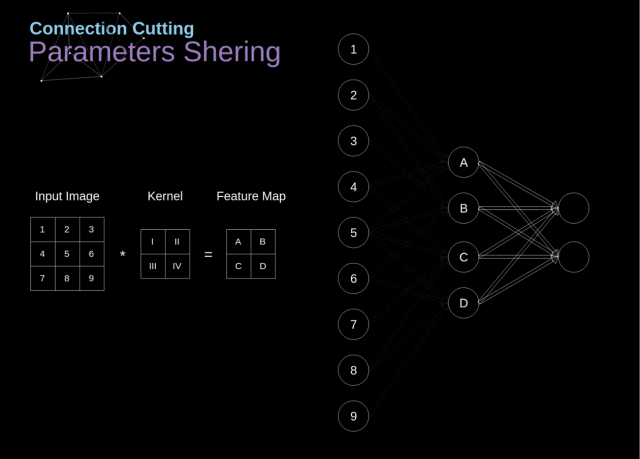Figure 9. Connections cutting and parameters sharing

Now, let's focus on the two convolutional layers A very important attribute. First, it can be seen from the figure that not all neurons in two adjacent layers are connected to each other. For example, neuron 1 only affects the value of A. Second, we can find that some neurons use the same weight. These two attributes mean that we have to learn a lot less parameters in CNN. It is worth mentioning that,Any single value in the kernel affects every element of the output feature map &mdash;&mdash; which is critical in the backpropagation process.

Convolutional Layer Backpropagation

Any attempt to start from scratch Anyone who started writing neural networks knows that forward propagation is less than half of the ultimate success. When you start to count back, the real fun is just beginning. Today, we don't need to worry about backpropagation &mdash;&mdash; because the deep learning framework is already done for us, but I think it's necessary to understand what's going on behind it. Just as in a densely connected neural network, our goal is to calculate the derivatives and then use these derivatives to update our parameter values as the gradient falls.

In the calculation below,We will use the chain rule &mdash;&mdash; which was mentioned in my previous article. We want to evaluate the effect of changes in the parameters on the resulting feature map and the subsequent impact on the final result. Before we start the detailed discussion, we need to unify the mathematical symbols &mdash;&mdash; For convenience, I will not use the complete notation of the partial derivative, but use the abbreviations mentioned below. But keep in mind that when I use this notation, this will always represent the partiality of the cost function.Figure 10. Input and output data for a single convolution layer in forward and backward propagation

Our task is to calculate dW  and db [l] (they are the derivatives associated with the current layer parameters) ), and dA[l-1] (which will be passed to the previous layer). As shown in Figure 10, dA[l] is used as input, tensors dW and W, db and b, and dA and A have the same dimensions The first step is to find the derivative of the activation function about the input tensor.Record the result as dZ  . According to the chain rule, the result of this operation will be used later.Now, we need to deal with the backpropagation of the convolution itself. To achieve this goal, we will use a matrix operation called full convolution, the visual interpretation of which is shown below. Note that we are going to use the kernel in this process, and the kernel we used earlier is the kernel rotated by 180 degrees. This operation can be expressed by the following formula, where kernel is represented by W and dZ[m,n] is a scalar.This scalar belongs to the partial derivative obtained from the previous layer.Pooling Layers

In addition to the convolutional layer, CNNs often use a network layer called the pooling layer, which is mainly used to reduce the size of the tensor and speed up the calculation. The structure of this layer is very simple, we only need to divide the image into different areas, and then perform some operations on each part. For example, for the Max Pool Layer, we select a maximum from each region and place it in the appropriate position in the output. As with the convolutional layer, we have two hyperparameters &mdash;&mdash;kernel size and step size. Finally, it is worth mentioning that if you want to perform pooling for multi-channel images, each channel should perform pooling operations separately.Figure 12. Max pooling example

Pooling Layers Backpropagation

In this article, we Only the maximum pooling backpropagation is discussed, but by slightly adjusting the method, it can be applied to all other types of pooling layers. Since this type of layer is not used in the pooling layer, we do not need To update any parameters, our task is to assign gradient values modestly. As mentioned earlier, in the maximum pooled forward propagation,We select the maximum from each region and transfer them to the next layer. It is therefore clear that during backpropagation, the gradient does not affect the matrix elements that are not used in forward propagation. In practice, the process is accomplished by creating a mask that remembers the position of the element used in forward propagation, which we can then use to pass the gradient.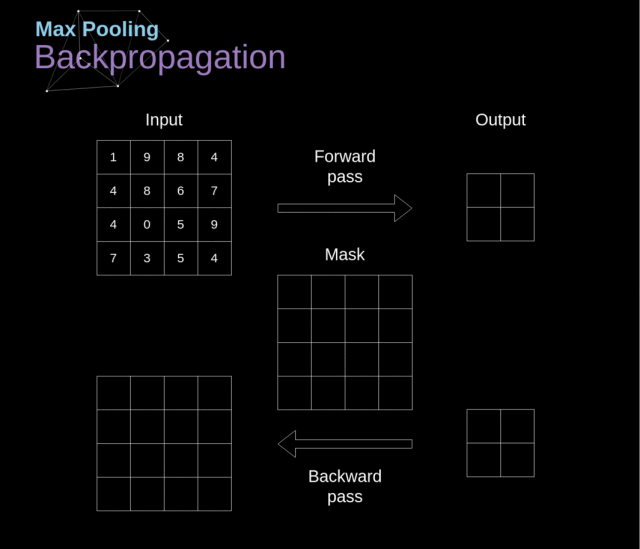Figure 13. Max pooling backward pass

Relevant articles recommend

Top suppliers

Product:Photo Coupler,Optocoupler,IC,TOS,ON,AVAGO,SHARP,RENESAS,CT,EL,LTV,TI,NXP,ST
1YRS
Product:Infrared Receiver Module,Infrared Emitting Diode,Silicon PIN Photodiode,Silicon Phototransistor,LED,Silicon Photocell,Hall Effect IC,Photoelectric switch,Photoelectric encoder,IC.
1YRS
Product:KSD301 KSD9700 thermostat thermal protector fuse sensor
1YRS
Product:IC,PCB,module,capacitor,resistor,diodes,LED,LCD
1YRS
Product:Electronics components，ICs，MCU，RS-485/RS-422 Transceiver
9YRS
Subscribe News